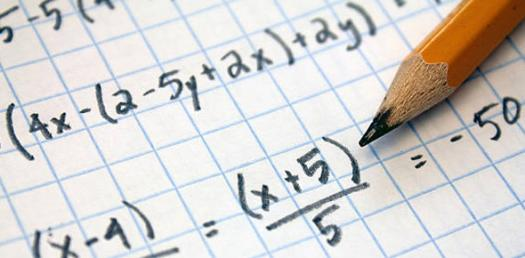# Algebra 1 Final Review Quiz

10 Questions | Attempts: 3565SettingsIf you are having a hard time understanding some algebra questions the quiz below is perfect for you. It has different algebraic questions for you to tackle. Give it a try and see if you can solve the problems correctly. All the best and remember that practice does make one perfect.

• 1.
What is the simplified form of this expression?8y+x-y
• A.

9y

• B.

7y-x

• C.

7y+x

• 2.
Which expression represents six less than four times a number?
• A.

6 - 4n

• B.

4n - 6

• C.

4/n - 6

• 3.
Find the volume of a figure with length of 4 feet, width of 6 feet, and height of 8 feet.
• A.

192 feet cubed

• B.

14 feet cubed

• C.

20 feet cubed

• 4.
A jacket originally cost \$75. It is on sale for \$50. What is the percent of discount for the jacket?
• A.

25%

• B.

33%

• C.

35%

• 5.
What is the mean of the following set of data?12, 12, 13, 14, 15, 18*remember mean = average add up ALL the numbers and divide by the total ( 6 )
• A.

12

• B.

14

• C.

13

• 6.
2 out of 6 is the same as:
• A.

4 out of 12

• B.

3 out of 7

• C.

5 out of 10

• 7.
Factor the following trinomial:x2 + 6x + 8
• A.

(x + 3) (x + 3)

• B.

(x + 2) (x + 4)

• C.

(x + 2) (x - 4)

• 8.
What is the slope of the following line?y = x + 4
• A.

4

• B.

0

• C.

1

• 9.
Which of the following equations has a negative slope?
• A.

Y = 4x - 3

• B.

Y = 4x + 3

• C.

Y = -4x + 3

• 10.
Y < 3Which of the following is true?
• A.

Y is equal to all numbers less than 3

• B.

Y is equal to all numbers less than or equal to 3

• C.

Y is greater than 3

## Related TopicsBack to top
×

Wait!
Here's an interesting quiz for you.